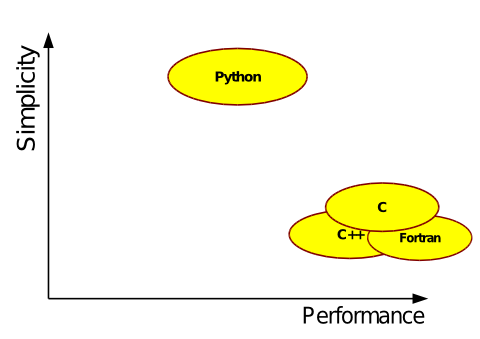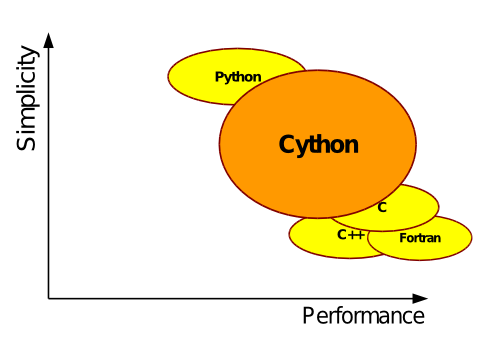# 用Cython编译写出更快的Python代码

2015/06/19 10:46

• Passionate Python developer since 2002

• after Basic, Logo, Pascal, Prolog, Scheme, Java, C, ...

• CS studies in Germany, Ireland, France

• PhD in distributed systems in 2007

• Language design for self-organising systems

• Darmstadt University of Technologies, Germany

• Current occupations:

• http://codespeak.net/lxml/

• IT transformations, SOA design, Java-Development, ...

• Employed by Senacor Technologies AG, Germany

• »lxml« OpenSource XML toolkit for Python

• »Cython«

# Part 1: Intro to Cython

• Part 1: Intro to Cython

• Part 2: Building Cython modules

• Part 3: Writing fast code

• Part 4: Talking to other extensions

# What is Cython?

between the simplicity of Python

and the speed of C / C++ / Fortran.# What is Cython?

between the simplicity of Python

and the speed of C / C++ / Fortran.# What is Cython?

Cython is

• an Open-Source project

• a Python compiler (almost)

• an enhanced, optimising fork of Pyrex

• an extended Python language for

• writing fast Python extension modules

• interfacing Python with C libraries

# Major Cython Core Developers

• Robert Bradshaw, Stefan Behnel, Dag Sverre Seljebotn

• Lisandro Dalcín

• C/C++ portability and various feature patches

• Kurt Smith, Danilo Freitas

• Google Summer of Code 2009: Fortran/C++ integration

• Greg Ewing

• main developer and maintainer of Pyrex

• many, many others - see

# How to use Cython

• you write Python code

• Cython translates it into C code

• your C compiler builds a shared library for CPython

• you import your module into CPython

• Cython has support for

• optionally compile Python code from setup.py!

• Cython does that for its own modules :-)

• distutils

• embedding the CPython runtime in an executable

# Example: compiling Python code

# file: worker.pyclass HardWorker(object):    u"Almost Sisyphos"    def __init__(self, task):        self.task = task    def work_hard(self, repeat=100):        for i in range(repeat):            self.task()def add_simple_stuff():
x = 1+1HardWorker(add_simple_stuff).work_hard()

• compile with

# Example: pyximport

Build and import Cython code files (.pyx) on the fly

$ls worker.pyx$ PYTHONPATH=. python

Python 2.6.2 (r262:71600, Apr 17 2009, 11:29:30)[GCC 4.3.2] on linux2Type "help", "copyright", "credits" or "license" for more information.>>> import pyximport>>> pyximport.install()>>> import worker>>> worker<module 'worker' from '~/.pyxbld/.../worker.so'>>>> worker.HardWorker<class 'worker.HardWorker'>>>> worker.HardWorker(worker.add_simple_stuff).work_hard()

# pyximporting Python modules

• pyximport can also compile Python modules:

>>> import pyximport>>> pyximport.install(pyimport = True)>>> import shlex[lots of compiler errors from different modules ...]>>> help(shlex)

• currently works for a few stdlib modules

• falls back to normal Python import automatically

• not production ready, but nice for testing :)

# Writing executable programs

# file: hw.pydef hello_world():    import sys    print "Welcome to Python %d.%d!" % sys.version_info[:2]if __name__ == '__main__':
hello_world()

# Writing executable programs

# file: hw.pydef hello_world():    import sys    print "Welcome to Python %d.%d!" % sys.version_info[:2]if __name__ == '__main__':
hello_world()

$cython --embed hw.py # <- embed a main() function$ gcc $CFLAGS -I/usr/include/python2.6 \ -o hw hw.c -lpython2.6 -lpthread -lm -lutil -ldl$ ./hw
Welcome to Python 2.6!

# Part 3: Writing fast code

• Part 1: Intro to Cython

• Part 2: Building Cython modules

• Part 3: Writing fast code

• Part 4: Talking to other extensions

# A simple example

• Plain Python code:

# integrate_py.pyfrom math import sindef f(x):    return sin(x**2)def integrate_f(a, b, N):
dx = (b-a)/N
s = 0    for i in range(N):
s += f(a+i*dx)    return s * dx

# Type declarations in Cython

Function arguments are easy

• Python:

def f(x):    return sin(x**2)
• Cython:

def f(double x):    return sin(x**2)

# Type declarations in Cython

»cdef« keyword declares

• variables with C or builtin types

cdef double dx, s
• functions with C signatures

cdef double f(double x):    return sin(x**2)
• classes as 'builtin' extension types

cdef class MyType:    cdef int field

# Functions: def vs. cdef vs. cpdef

• def func(int x):

• part of the Python module API

• Python call semantics

• cdef int func(int x):

• C signature

• C call semantics

• cpdef int func(int x):

• Python wrapper around cdef function

• C calls cdef function, Python calls wrapper

• note: modified C signature!

# Typed arguments and return values

• def func(int x):

• caller passes Python objects for x

• function converts to int on entry

• implicit return type always object

• cdef int func(int x):

• caller converts arguments as required

• function receives C int for x

• arbitrary return type, defaults to object

• cpdef int func(int x):

• wrapper converts

• C callers convert arguments as required

• Python callers pass and receive objects

# A simple example: Python

# integrate_py.pyfrom math import sindef f(x):    return sin(x**2)def integrate_f(a, b, N):
dx = (b-a)/N
s = 0    for i in range(N):
s += f(a+i*dx)    return s * dx

# A simple example: Cython

# integrate_cy.pyxcdef extern from "math.h":
double sin(double x)cdef double f(double x):    return sin(x**2)cpdef double integrate_f(double a, double b, int N):    cdef double dx, s    cdef int i

dx = (b-a)/N
s = 0    for i in range(N):
s += f(a+i*dx)    return s * dx

# Overriding declarations in .pxd

• Plain Python code:

# integrate_py.pyfrom math import sindef f(x):    return sin(x**2)def integrate_f(a, b, N):
dx = (b-a)/N
s = 0    for i in range(N):
s += f(a+i*dx)    return s * dx

# Overriding declarations in .pxd

 Python integrate_py.py Cython integrate_py.pxd # integrate_py.pyfrom math import sindef f(x):    return sin(x**2)def integrate_f(a, b, N):     dx = (b-a)/N     s = 0    for i in range(N):         s += f(a+i*dx)    return s * dx # integrate_py.pxdcimport cythoncpdef double f(double x)@cython.locals(     dx=double, s=double, i=int)cpdef integrate_f(     double a, double b, int N)

# The .pxd file used

# integrate_py.pxdcimport cythoncpdef double f(double x):    return sin(x**2)cpdef double integrate_f(double a, double b, int N)

# Overriding declarations in .pxd

• Eclipse, pylint, 2to3, ...

• runs unchanged in Python interpreter

• plain Python code

• complete Python tool-chain available

• drawback:

• cannot override from math import sin

# Typing in Python syntax

• Plain Python code:

# integrate_py.pyfrom math import sindef f(x):    return sin(x**2)def integrate_f(a, b, N):
dx = (b-a)/N
s = 0    for i in range(N):
s += f(a+i*dx)    return s * dx

# Typing in Python syntax

from math import sinimport cython@cython.locals(x=cython.double)def f(x):    return sin(x**2)@cython.locals(a=cython.double, b=cython.double,
N=cython.Py_ssize_t, dx=cython.double,
s=cython.double, i=cython.Py_ssize_t)def integrate_f(a, b, N):
dx = (b-a)/N
s = 0    for i in range(N):
s += f(a+i*dx)    return s * dx

# Declaring Python types

• for ... in range()/list/tuple/dict

• list.append(), list.reverse()

• set([...]), tuple([...])

• Further improvements in Cython 0.12

• replacements for enumerate(), type()

• dict([...]), unicode.encode(), list.sort()

• Declaring Python types is often worth it!

• Easy to add new optimisations

• don't write prematurely optimised code, fix Cython!

# Declaring Python types: dict

• example: dict iteration

def filter_a(d):    return { key : value             for key, value in d.iteritems()             if 'a' not in value }import stringd = { s:s for s in string.ascii_letters }print filter_a(d)

# Declaring Python types: dict

• simple change, ~30% faster:

def filter_a(dict d):       # <====    return { key : value             for key, value in d.iteritems()             if 'a' not in value }import stringd = { s:s for s in string.ascii_letters }print filter_a(d)

# Declaring Python types: dict

• simple change, ~30% faster:

def filter_a(dict d):       # <====    return { key : value             for key, value in d.iteritems()             if 'a' not in value }import stringd = { s:s for s in string.ascii_letters }print filter_a(d)

• drawback:

• non-dict mapping arguments raise a TypeError

# Think twice before you type• benchmark code before adding static types!

# Classes

• class MyClass(object):

• Python class with __dict__

• multiple inheritance

• arbitrary Python attributes

• Python methods

• monkey-patcheable etc.

• cdef class MyClass(SomeSuperClass):

• C-only access by default, or readonly/public

• only from other extension types!

• "builtin" extension type

• single inheritance

• fixed, typed fields

• Python + C methods

# cdef classes - when to use them?

• Use cdef classes

• e.g. whenever wrapping C structs/pointers/etc.

• when C attribute types are used

• when the need for speed beats Python's generality

• Use Python classes

• for bytes/tuple subtypes (PyVarObject)

• for exceptions if Py<2.5 compatibility is required

• when multiple inheritance is required

• when users are allowed to monkey-patch

# Part 4: Talking to other extensions

• Part 1: Intro to Cython

• Part 2: Building Cython modules

• Part 3: Writing fast code

• Part 4: Talking to other extensions

# Talking to other extensions

• Python 3 buffer protocol (available in Py2.6)

• external C-APIs

# Python 3 buffer protocol

• Native support for new Python buffer protocol

• PEP 3118

def inplace_invert_2D_buffer(                object[unsigned char, 2] image):    cdef int i, j    for i in range(image.shape):        for j in range(image.shape):
image[i, j] = 255 - image[i, j]

• can be supported for extension types in Py2.x

• declared through .pxd files

• Cython ships with numpy.pxd

• array.pxd available (stdlib's array)

# Conclusion

• Cython is a tool for

• translating Python code to efficient C

• easily interfacing to external C/C++/Fortran code

• Use it to

• concentrate on the mapping, not the glue!

• don't change the language just to get fast code!

• concentrate on optimisations, not rewrites!

• speed up existing Python modules

• write C extensions for CPython

• wrap C libraries in Python

# ... but Cython is also

• a great project

• a very open playground for great ideas!

# Cython

Cython

C-Extensions in Python

... use it, and join the project!

http://cython.org/

0
8 收藏

### 作者的其它热门文章0 评论
8 收藏
0